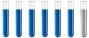## General chemistry question

Chemistry and homework help forum.

Organic Chemistry, Analytical Chemistry, Biochemistry, Physical Chemistry, Computational Chemistry, Theoretical Chemistry, High School Chemistry, Colledge Chemistry and University Chemistry Forum.

Share your chemistry ideas, discuss chemical problems, ask for help with scientific chemistry questions, inspire others by your chemistry vision!

Please feel free to start a scientific chemistry discussion here!

Discuss chemistry homework problems with experts!

Ask for help with chemical questions and help others with your chemistry knowledge!

Moderators: expert, ChenBeier, Xen

Dhamnekar Winod
Sr. Staff MemberPosts: 118
Joined: Sat Nov 21, 2020 10:14 am
Location: Mumbai[Bombay],Maharashtra State,India

### General chemistry question

How to compute $$\Delta H^\circ, \Delta S^\circ, \Delta G^\circ$$ at $$25^\circ C$$ for the following reactions?

1)Combustion of Methane

2)Formation of gaseous HCl from its elements.

3)Formation of $$H_3SiCl$$ from its elements [ We will need to use bond energies and estimate $$\Delta S^\circ]$$

My Answers: I know the reaction equations for 1)$$CH_4 (g) + 2O_2(g) \rightarrow CO_2(g) + 2H_2O(l)$$

2)$$Cl_2(g)+ H_2(g)\rightarrow 2HCl(g)$$
3) $$Si(s) + 3HCl(aq) \rightarrow H_3SiCl(g) + Cl_2(g)$$

$$\Delta H^\circ_{rxn}$$(Enthalpy of reaction) for first reaction is -393.5 kJ/mol -285.8 kJ/mol-(-74.6 kJ/mol)=-604.7 kJ/mol Reaction is exothermic.

$$\Delta H^\circ_{rxn}$$ (Enthalpy of reaction) for second reaction is -92.3 kJ/mol Reaction is exothermic.

$$\Delta S^\circ_{rxn}$$ Spontaneity for the first reaction is 213.8 J/mol K+ 140 J/mol K - 186.3 J/mol K -410.4 J/mol K = -242.9 J/mol K First reaction is spontaneous at low temperature.

$$\Delta S^\circ_{rxn}$$ Spontaneity for the second reaction is 373.8 J/mol K - 223.1 J/mol K -130.7 J/mol K=20 J/mol K Second reaction is spontaneous at all temperature.

Gibbs free energy for the first reaction's $$( \Delta G^\circ_r)$$ is -604.7 kJ/mol - 298 k * (-242.9 J/mol K) =-532.32 kJ/mol

Gibbs free energy for the second reaction ($$\Delta G^\circ_r)$$ is -92.3 kJ/mol- 298 K*(20.0 J/mol K)= -86.34 kJ/mol

Enthalpy of third reaction $$(\Delta H^\circ_f)$$ = -141.84 kJ/mol- (-501.6 kJ/mol)=359.76 kJ/mol This reaction is endothermic.

$$\Delta S^\circ_r$$ for the third reaction is 250.76 J/mol K -188.3 J/mol K = 62.46 J/mol K The third reaction is non-spontaneous at low temperature.

$$\Delta G^\circ_r$$ for the third reaction is 359.76 kJ/mol - 298 K*(62.46 J/mol K)=341.15 kJ/mol

Are my computations of $$\Delta H^\circ_f, \Delta S^\circ_r, \Delta G^\circ_r$$ for all these three reactions correct?
Any science consists of the following process. 1) See 2)Hear 3)Smell,if needed 4)Taste, if needed 5)Think 6)Understand 7)Inference 8)Take decision [Believe or disbelieve, useful or useless, true or false, cause or effect, any other criteria]